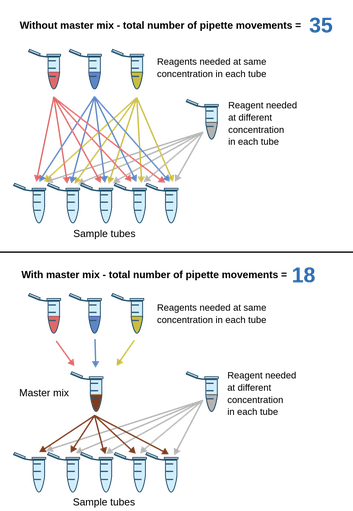For an experiment with 7 sample tubes and 5 reagents (4 of which are required at the same concentration), the number of required pipette movements are shown. The figure below shows the number of pipette movements necessary without the use of a master mix. For the same experiment, a master mix is now used. Notice the drop in the number of pipette movements - imagine even larger experiments and the time and workload difference achieved.Figure 1: Two experimental setups with 7 sample tubes and 5 reagents (4 of which are required at the same concentration). The number of required pipette movements is shown. This figure shows the number of pipette movements necessary with and without the use of a master mix.

When conducting an experiment with a large number of similar samples, it is common to prepare a master mix to ease the workload in the lab. If all your samples have a similar mixture of reagents with only 1 reagent varying from sample to sample, a master mix can be prepared with all the common reagents (of course, this is only possible if all reagents are required in the same concentrations). Now, when setting up your samples, you can pipette from the master mix and then add your variable reagent, instead of mixing e.g., 4 different reagents in correct amounts into each and every tube. The concept is illustrated in the figure above.

In this enzyme kinetics case, at a specific substrate concentration, a master mix, containing NAD+, ethanol, and buffer, can be prepared. When the enzyme is added, the reaction starts, and the enzyme is therefore not included in the master mix. This is not a case where it is crucial to use a master mix; however, the skill is essential for many applications, and it is very useful in enzyme kinetics assays in general.

## Calculating substrate concentrations

In the experiment, you will need to calculate how much substrate to add to a tube to obtain the desired substrate concentration. This can be done using the following formula:

C1 · V1 = C2 · V2

where C = concentration and V = volume. Remember to use the same units on both sides of the equality sign!

Example: We have a stock solution of the substrate at 1 M, and we need a final concentration of 200 mM in a volume of 500 μl; what is the volume of stock solution that we need to add for achieving the desired concentration?

If the stock concentration is C1, C2 will be the desired final concentration and V2 will be the final volume. This makes V1 the unknown element. We can now isolate V1, insert the known values and calculate the volume:

Isolate: V1 = (C2 · V2)/C1

Insert and calculate: V1 = (0.2 M · 0.0005l) / 1 M = 0.0001 L = 100 μl

Theory overview